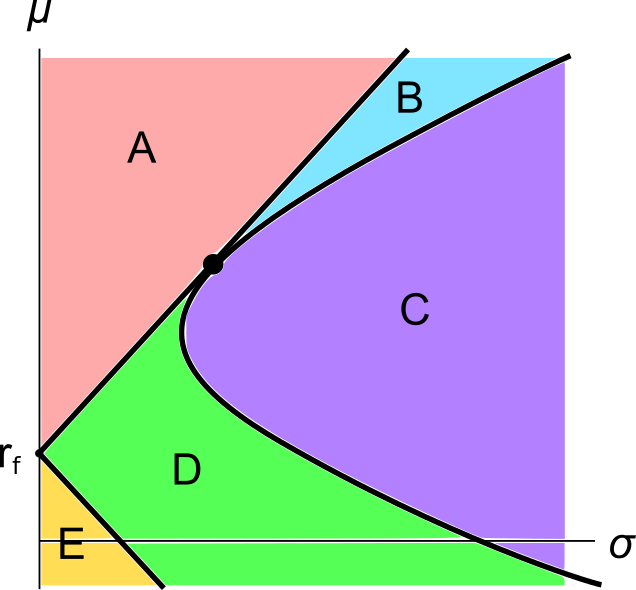# Fight Finance

#### CoursesTagsRandomAllRecentScores

A graph of assets’ expected returns $(\mu)$ versus standard deviations $(\sigma)$ is given in the below diagram.

Each letter corresponds to a separate coloured area. The portfolios at the boundary of the areas, on the black lines, are excluded from each area. Assume that all assets represented in this graph are fairly priced, and that all risky assets can be short-sold.

Which of the following statements about this graph and Markowitz portfolio theory is NOT correct?A graph of assets’ expected returns $(\mu)$ versus standard deviations $(\sigma)$ is given in the graph below. The CML is the capital market line.Which of the following statements about this graph, Markowitz portfolio theory and the Capital Asset Pricing Model (CAPM) theory is NOT correct?